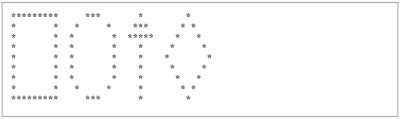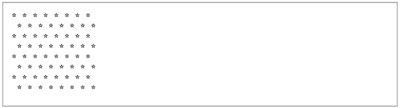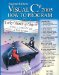# Wrap-Up

### Answers to Self Review Exercises

3.1

a) left brace ({), right brace (}). b) semicolon (;). c) if. d) //. e) Blank lines, space characters, tab characters. f) Keywords. g) Main. h) Console.WriteLine and Console.Write.

3.2
1. False. Comments do not cause any action to be performed when the application executes. They are used to document applications and improve their readability.
2. True.
3. False. C# is case sensitive, so these variables are distinct.
4. False. The remainder operator also can be used with noninteger operands in C#.
5. False. The operators *, / and % are on the same level of precedence, and the operators + and - are on a lower level of precedence.
3.3
1. int c, thisIsAVariable, q76354, number;

or

```int c;
int thisIsAVariable;
int q76354;
int number;
```
2. Console.Write( "Enter an integer: " );
3. value = Convert.ToInt32( Console.ReadLine() );
4. if ( number != 7 ) Console.WriteLine( "The variable number is not equal to 7" );
5. Console.WriteLine( "This is a C# application" );
6. Console.WriteLine( "This is a C# application" );
7. Console.WriteLine( "{0} {1}", "This is a C#", "application" );
3.4

The solutions to Self-Review Exercise 3.4 are as follows:

1. Error: Semicolon after the right parenthesis of the condition ( c < 7 ) in the if statement.

Correction: Remove the semicolon after the right parenthesis. [Note: As a result, the output statement will execute regardless of whether the condition in the if is true.]

2. Error: The relational operator => is incorrect. Correction: Change => to >=.
3.5
1. // Calculate the product of three integers
2. int x, y, z, result;

or

```int x;
int y;
int z;
int result;
```
3. Console.Write( "Enter first integer: " );
4. x = Convert.ToInt32( Console.ReadLine() );
5. Console.Write( "Enter second integer: " );
6. y = Convert.ToInt32( Console.ReadLine() );
7. Console.Write( "Enter third integer: " );
8. z = Convert.ToInt32( Console.ReadLine() );
9. result = x * y * z;
10. Console.WriteLine( "Product is {0}", result );
3.6

The solution to Exercise 3.6 is as follows:

``` 1 // Exercise 3.6: Product.cs
2 // Calculating the product of three integers.
3 using System;
4
5 public class Product
6 {
7 public static void Main( string[] args )
8 {
9 int x; // declare first number to be entered by user
10 int y; // declare second number to be entered by user
11 int z; // declare third number to be entered by user
12 int result; // product of numbers
13
14 Console.Write( "Enter first integer: " ); // prompt for input
16
17 Console.Write( "Enter second integer: " ); // prompt for input
19
20 Console.Write( "Enter third integer: " ); // prompt for input
22
23 result = x * y * z; // calculate the product of the numbers
24
25 Console.WriteLine( "Product is {0}", result );
26 } // end method Main
27 } // end class Product
```

 ```Enter first integer: 10 Enter second integer: 20 Enter third integer: 30 Product is 6000 ```

### Exercises

3.7

Fill in the blanks in each of the following statements:

1. __________ are used to document an application and improve its readability.
2. A decision can be made in a C# application with a(n) __________.
3. Calculations are normally performed by __________ statements.
4. The arithmetic operators with the same precedence as multiplication are __________ and __________.
5. When parentheses in an arithmetic expression are nested, the __________ set of parentheses is evaluated first.
6. A location in the computer's memory that may contain different values at various times throughout the execution of an application is called a(n) __________.
3.8

Write C# statements that accomplish each of the following tasks:

1. Display the message "Enter an integer: ", leaving the cursor on the same line.
2. Assign the product of variables b and c to variable a.
3. State that an application performs a sample payroll calculation (i.e., use text that helps to document an application).

3.9

State whether each of the following is true or false. If false, explain why.

1. C# operators are evaluated from left to right.
2. The following are all valid variable names: _under_bar_, m928134, t5, j7, her_sales, his_account_total, a, b, c, z and z2.
3. A valid C# arithmetic expression with no parentheses is evaluated from left to right.
4. The following are all invalid variable names: 3g, 87, 67h2, h22 and 2h.
3.10

Assuming that x = 2 and y = 3, what does each of the following statements display?

1. Console.WriteLine( "x = {0}", x );
2. Console.WriteLine( "Value of {0} + {0} is {1}", x, ( x + x ) );
3. Console.Write( "x =" );
4. Console.WriteLine( "{0} = {1}", ( x + y ), ( y + x ) );
3.11

Which of the following C# statements contain variables whose values are modified?

1. p = i + j + k + 7;
2. Console.WriteLine( "variables whose values are modified" );
3. Console.WriteLine( "a = 5" );
4. value = Convert.ToInt32( Console.ReadLine() );
3.12

Given that y = ax3 + 7, which of the following are correct C# statements for this equation?

1. y = a * x * x * x + 7;
2. y = a * x * x * ( x + 7 );
3. y = ( a * x ) * x * ( x + 7 );
4. y = ( a * x ) * x * x + 7;
5. y = a * ( x * x * x ) + 7;
6. y = a * x * ( x * x + 7 );
3.13

State the order of evaluation of the operators in each of the following C# statements and show the value of x after each statement is performed:

1. x = 7 + 3 * 6 / 2 - 1;
2. x = 2 % 2 + 2 * 2 - 2 / 2;
3. x = ( 3 * 9 * ( 3 + ( 9 * 3 / ( 3 ) ) ) );
3.14

Write an application that displays the numbers 1 to 4 on the same line, with each pair of adjacent numbers separated by one space. Write the application using the following techniques:

1. Use one Console.WriteLine statement.
2. Use four Console.Write statements.
3. Use one Console.WriteLine statement with four format items.
3.15

Write an application that asks the user to enter two integers, obtains them from the user and prints their sum, product, difference and quotient (division). Use the techniques shown in Fig. 3.18.

3.16

Write an application that asks the user to enter two integers, obtains them from the user and displays the larger number followed by the words "is larger". If the numbers are equal, print the message "These numbers are equal." Use the techniques shown in Fig. 3.26.

3.17

Write an application that inputs three integers from the user and displays the sum, average, product, and smallest and largest of the numbers. Use the techniques shown in Fig. 3.26. [Note: The calculation of the average in this exercise should result in an integer representation of the average. So if the sum of the values is 7, the average should be 2, not 2.3333....]

3.18

Write an application that displays a box, an oval, an arrow and a diamond using asterisks (*), as follows:3.19

What does the following code print?

```Console.WriteLine( "*
**
***
****
*****" );
```
3.20

What does the following code print?

```Console.WriteLine( "*" );
Console.WriteLine( "***" );
Console.WriteLine( "*****" );
Console.WriteLine( "****" );
Console.WriteLine( "**" );
```
3.21

What does the following code print?

```Console.Write( "*" );
Console.Write( "***" );
Console.Write( "*****" );
Console.Write( "****" );
Console.WriteLine( "**" );
```
3.22

What does the following code print?

```Console.Write( "*" );
Console.WriteLine( "***" );
Console.WriteLine( "*****" );
Console.Write( "****" );
Console.WriteLine( "**" );
```
3.23

What does the following code print?

```Console.WriteLine( "{0}
{1}
{2}", "*", "***", "*****" );
```
3.24

Write an application that reads five integers, then determines and prints the largest and smallest integers in the group. Use only the programming techniques you learned in this chapter.

3.25

Write an application that reads an integer and determines and prints whether it is odd or even. [Hint: Use the remainder operator. An even number is a multiple of 2. Any multiple of 2 leaves a remainder of 0 when divided by 2.]

3.26

Write an application that reads two integers, determines whether the first is a multiple of the second and prints the result. [Hint: Use the remainder operator.]

3.27

Write an application that displays a checkerboard pattern, as follows:3.28

Here's a peek ahead. In this chapter, you have learned about integers and the type int. C# can also represent floating-point numbers that contain decimal points, such as 3.14159. Write an application that inputs from the user the radius of a circle as an integer and prints the circle's diameter, circumference and area using the floating-point value 3.14159 for p. Use the techniques shown in Fig. 3.18. [Note: You may also use the predefined constant Math.PI for the value of p. This constant is more precise than the value 3.14159. Class Math is defined in namespace System. Use the following formulas (r is the radius):

diameter = 2r

circumference = 2pr

area = pr2

Do not store the results of each calculation in a variable. Rather, specify each calculation as the value that will be output in a Console.WriteLine statement. Note that the values produced by the circumference and area calculations are floating-point numbers. You will learn more about floating-point numbers in Chapter 4.

3.29

Here's another peek ahead. In this chapter, you have learned about integers and the type int. C# can also represent uppercase letters, lowercase letters and a considerable variety of special symbols. Every character has a corresponding integer representation. The set of characters a computer uses and the corresponding integer representations for those characters is called that computer's character set. You can indicate a character value in an application simply by enclosing that character in single quotes, as in 'A'.

You can determine the integer equivalent of a character by preceding that character with (int), as in

```(int) 'A'
```

The keyword int in parentheses is known as a cast operator, and the entire expression is called a cast expression. (You will learn about cast operators in Chapter 5.) The following statement outputs a character and its integer equivalent:

```Console.WriteLine( "The character {0} has the value {1}",
'A', ( ( int ) 'A' ) );
```

When the preceding statement executes, it displays the character A and the value 65 (from the Unicode® character set) as part of the string.

Using statements similar to the one shown earlier in this exercise, write an application that displays the integer equivalents of some uppercase letters, lowercase letters, digits and special symbols. Display the integer equivalents of the following: A B C a b c 0 1 2 \$ * + / and the blank character.

3.30

Write an application that inputs one number consisting of five digits from the user, separates the number into its individual digits and prints the digits separated from one another by three spaces each. For example, if the user types in the number 42339, the application should print

 4 2 3 3 9

Assume that the user enters the correct number of digits. What happens when you execute the application and type a number with more than five digits? What happens when you execute the application and type a number with fewer than five digits? [Hint: It is possible to do this exercise with the techniques you learned in this chapter. You will need to use both division and remainder operations to "pick off" each digit.]

3.31

Using only the programming techniques you learned in this chapter, write an application that calculates the squares and cubes of the numbers from 0 to 10 and prints the resulting values in table format, as shown below. All calculations should be done in terms of a variable x. [Note: This application does not require any input from the user.]

 number square cube 0 0 0 1 1 1 2 4 8 3 9 27 4 16 64 5 25 125 6 36 216 7 49 343 8 64 512 9 81 729 10 100 1000
3.32

Write an application that inputs five numbers and determines and prints the number of negative numbers input, the number of positive numbers input and the number of zeros input.Visual C# 2005 How to Program (2nd Edition)
ISBN: 0131525239
EAN: 2147483647
Year: 2004
Pages: 600